## Conservation of Linear Momentum

### Conservation of Linear Momentum### Conservation of Linear Momentum

Almost everybody can easily stop a ball thrown at 40 km/h in a tenth of a second but nobody can do the same with a car travelling at 40 km/h.

It’s the product of mass and change in velocity that really matters when it comes to evaluating the forces exerted on a body, in order to change its motion.

Definition of momentum

It is convenient to define a physical quantity called momentum, usually indicated by the symbol, as the product of its massby its velocity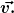The momentum is a vector quantity which has the same direction as the velocity of a body.
The unit of measurement of momentum in the SI system is the product of the units of mass and velocity and thus is kg m/s.

A new Form of Newton’s Second Law

Newton’s Second Law can be expressed in terms of change in momentum:which can be written in the more compact formIt’s interesting to notice that this is actually the way in which Newton wrote his second law in his original work Philosophiae Naturalis Principia Mathematica (Latin for “Mathematical Principles of Natural Philosophy”).

Since we can write this equation as(a simple idea which, as we will see later, has very interesting consequences), the SI units of momentum should be equivalent to the product of the units of force and time and this is indeed the case as kg m/s = N s.

Example 1

If a constant net force of 130 N acts for 10 ms on a 50 g ball initially at rest, what will its final momentum be?

Since the ball is initially at rest, its initial momentum is zero:. From the expression of Newton’s Second Law we getThe final momentum will have thus the same direction as the force acting on the body and magnitude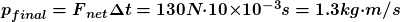The impulse of a force and its graphical interpretation

In the expressionthe net force on the left-hand side is the average force acting during the time. We can give a precise meaning to this average force by introducing another physical quantity named the impulse.

The impulse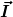of a constant forceacting for a timeis given byThe impulse is a vector quantity. For a constant force, its direction is the same as the direction of the force. The SI unit of impulse is N s.

If we plot the intensity of the force versus time, for a constant force the graph will look like the one below:The magnitude of the impulse is represented by the area highlighted in yellow.

We can represent the magnitude of the variable force on a plot as below:The impulse delivered by the variable force has the same direction of the force and, like in the case of a constant force, has intensity equal to the area below the graph of the intensity of the force vs the t axis.

Example 2
The magnitude of a force of constant direction varies with time according to the plot below. What is the impulse of the force?The impulse and the force have the same direction. To know the magnitude of the impulse, we need to calculate the area of the triangle. The base is 0.8 s while the height is 8.0 N. So the magnitude of the impulse is given byAverage force
The definition of impulse of a variable force allows us to give a precise definition of average force.

If a variable forceacts for a time, its average valueis the value of a constant force that delivers the same impulseas the forcein the same time: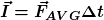For a variable force of constant direction, the average value of the magnitude is such that, in a force versus time plot like the one below, the area below the green line equals the area below the yellow line:Impulse and momentum change

Newton’s Second Law in the formcan be easily rewritten asSince theon the left-hand side is actually the average force, the whole left-hand side is the impulse of the net force. Hence, we can write that the impulse of the net force acting on a body is equal to its change in momentum:Example 3

A 50 g tennis ball is falling vertically to the ground. Immediately before hitting the ground its speed is 15 m/s. Immediately after the bounce its speed is 11 m/s. The ground contact time is 10 ms. What is the average normal force exerted by the ground on the ball?

Let’s start by calculating the impulse of the net force, which is easily obtained in terms of the change in momentum.Since the velocity changes direction, so too does the momentum. The momentum change is directed upwards and its magnitude is given by the sum of the magnitudes of the initial and final momentumThe average net force during contact is also directed upwards and its magnitude is given by the magnitude of the impulse divided by the contact timeDuring contact two forces act on the ball: the weightand the normal forceexerted by the ground. The net force is just the vector sum of these two. Since the weight is constant, its average is just its value:Since the net force is directed upwards and the weight force downwards, the magnitude of their difference is the sum of their magnitudes. Considering the average values, we haveMomentum of a system

In the example we have just discussed, the weight force is much less than the contact force between the ball and the ground. This is very common in case of rebounds, collisions or explosions: the contact force between the bodies involved are much larger than any other force involved.

When a system is composed of several bodies, the total momentum of the systemis simply the sum of the momenta of the bodies the system is made up of:Internal and external forces

We can distinguish the forces acting on each body of the system in two categories: internal and external. Internal forces are exerted by other bodies of the same system, while external forces are exerted by bodies which are not part of the system.

There is a very important result regarding internal forces.

The vector sum of all the internal forces acting in a system is null.

This is a direct consequence of Newton’s third law.

Let’s consider two bodies A and B inside our system. Newton’s third law states that if there is a forcethat A exerts on B, then there is also a forcethat B exerts on A and.

Thus if we add all internal forces, we need to put into the sum bothand. Since they are opposite, their sum isThis is true for any force exerted by a body of the system on any other body of the same system and thus the internal forces always come in couples whose sum is null. Hence, the sum of all internal forces in null.

Conservation of momentum

Let’s now consider the change in total momentumin a time intervalduring which both internal and external forces act on the bodies of the system. It will be equal to the sum of the momentum changes of each body:The momentum of a body of the system will change due to the effect of both internal and external forces acting on it. If we callandrespectively the sum of all internal and all external forces, we getSince we have already proven that the sum of all internal forces is zero,, we getFrom this analysis we can deduce a very important result, known as the law of conservation of momentum.

If the sum of all external forces acting on a system is null, then its total momentum doesn’t change:Collisions and explosions
In a collision or an explosion, the forces acting on the bodies involved are much larger than the external forces. Often, the sum of all external forces is zero but even when it is not so, they are so much weaker than the internal forces that they can be neglected. In such situations, we can apply the law of conservation of momentum.

Example 4

A cart of mass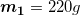is moving on a straight rail towards another cart of masswhich is initially at rest. The initial speed of the first cart is. After the collision, the two carts remain attached to each other. What will be the final speed of the two carts?

Let’s callthe final speed of both carts. Applying the conservation of momentum we getSincewe getElastic, inelastic and explosive collisions

Collisions can be distinguished in three categories, according to what happens to the total kinetic energy of the bodies involved.

For a system composed of multiple bodies, the total kinetic energy is simply the sum of the kinetic energies of all its bodies. If a body of massis point-like or doesn’t spin during its motion, and has speed, its kinetic energy is given byThe total kinetic energy of a system of bodies is thus given byWe can distinguish collisions in three categories

Elastic collisions: the total kinetic energy of the system doesn’t changeInelastic collisions: the final total kinetic energy is less than the initial total kinetic energy